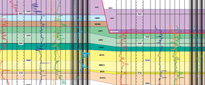# Geotechnical data | Attribute descriptions

COLUMN NAME DATA TYPE DESCRIPTION
GEOTECHNICAL_DATA_ID NUMBER Unique identifier of a geotechnical data record.
ACTIVITY_ID NUMBER Unique identifier of a sampling activity.
SAMPLE_NAME TEXT Unique name used to identify a sample/activity. It is a concatenation of the survey station identifier, the equipment code and the equipment attempt.
TERMS_OF_USE TEXT Terms under which the data can be used.
TERMS_OF_USE_URL TEXT Location of the terms of use.
SAMPLE_ALIAS TEXT Alternative name by which an activity may be identified. May have been used in literature or by an external organisation.
SAMPLE_SOURCE TEXT Cruise or Offshore Report with which the activity is associated.
CLIENT TEXT If activity is linked to a cruise, client for whom the project was carried out. If activity is linked to a report, client for whom the report was produced.
CONTRACTOR TEXT If activity is linked to a cruise, contractor who undertook the project on behalf of the client. If activity is linked to a report, contractor who produced the report on behalf of the client.
EQUIPMENT_TYPE TEXT Type of equipment used for sampling.
EQUIPMENT_START_DATE DATE Date on which use of a specific piece of equipment began.
EPSG_CODE NUMBER EPSG (OGP) code for the horizontal coordinate system.
EPSG TEXT Translation of the EPSG (OGP) code for the horizontal coordinate system.
X NUMBER The X coordinate. It may be lat/long, national grid, UTM, etc.
Y NUMBER The Y coordinate. It may be lat/long, national grid, UTM, etc.
XY_SOURCE TEXT Source of the X and Y coordinates. It should normally be the original value but may have been recalculated to give better accuracy.
X_ED50 NUMBER X coordinate using the ED50 coordinate system.
Y_ED50 NUMBER Y coordinate using the ED50 coordinate system.
X_WGS84 NUMBER X coordinate using the WGS84 coordinate system.
Y_WGS84 NUMBER Y coordinate using the WGS84 coordinate system.
X_BNG NUMBER Easting (X) using the British National Grid coordinate system.
Y_BNG NUMBER Northing (Y) using the British National Grid coordinate system.
DEPTH_UNITS TEXT Depth units, metres (to the nearest centimetre).
WATER_DEPTH NUMBER Water depth in metres.
DEPTH_DATUM TEXT Depth datum (vertical coordinate system).
DEPTH_SOURCE TEXT Source of the water depth.
TERMINAL_DEPTH NUMBER Maximum depth achieved by an activity in metres (to the nearest centimetre).
MEAS_DEPTH NUMBER Depth of reading relative to the seabed in metres (to the nearest centimetre).
MEAS_SOURCE TEXT Source of the measurement.
MEAS_ORIENTATION TEXT Measurement orientation (Vertical, Horizontal or Unknown).
SHEAR_STRENGTH NUMBER Shear strength, the average of the hand vane readings multiplied by the conversion factor (in kPa).
SHEAR_STRENGTH_UNITS TEXT Units of measurement for SHEAR_STRENGTH, kPa.
COMPR_STRENGTH NUMBER Compressive strength, the average of the penetrometer head readings multiplied by the conversion factor (in kPa).
COMPR_STRENGTH_UNITS TEXT Units of measurement for COMPR_STRENGTH, kPa.
MOISTURE_CONTENT NUMBER Ratio of the mass of water to the mass of solids, expressed as a percentage.
MOISTURE_CONTENT_UNITS TEXT Units of measurement for MOISTURE_CONTENT, Percent.
PLASTIC_LIMIT NUMBER Moisture content where the thread breaks apart at a diameter of 3.2 mm (about 1/8 inch), expressed as a percentage.
PLASTIC_LIMIT_UNITS TEXT Units of measurement for PLASTIC_LIMIT, Percent.
LIQUID_LIMIT NUMBER Liquid limit (LL) is conceptually defined as the water content at which the behavior of a clayey soil changes from plastic to liquid, expressed as percentage.
LIQUID_LIMIT_UNITS TEXT Units of measurement for LIQUID_LIMIT, Percent.
PLASTICITY_INDEX NUMBER Plasticity index (PI) is a measure of the plasticity of a soil. The plasticity index is the size of the range of water contents where the soil exhibits plastic properties, expressed as percentage.
PLASTICITY_INDEX_UNITS TEXT Units of measurement for PLASTICITY_INDEX, Percent.
SOIL_ACTIVITY NUMBER Plasticity index divided by the percent of clay–sized particles (less than 2 um) present. Different types of clays have different specific surface areas which controls how much wetting is required to move a soil from one phase to another such as across the liquid limit or the plastic limit. From the activity, one can predict the dominant clay type present in a soil sample.
LIQUIDITY_INDEX NUMBER Liquidity index (LI) is used for scaling the natural water content of a soil sample to the limits.
BULK_DENSITY NUMBER Total mass of air, water, solids divided by the total volume of air water and solids, expressed as Mg/m3.
BULK_DENSITY_UNITS TEXT Units of measurement for BULK_DENSITY, Mg/m3.
DRY_DENSITY NUMBER Mass of solids divided by the total volume of air water and solids, expressed as Mg/m3.
DRY_DENSITY_UNITS TEXT Units of measurement for DRY_DENSITY, Mg/m3.
SATURATED_DENSITY NUMBER Bulk density of the soil when it is fully saturated, expressed as Mg/m3.
SATURATED_DENSITY_UNITS TEXT Units of measurement for SATURATED_DENSITY, Mg/m3.
VOIDS_RATIO NUMBER Ratio of the volume of voids to the volume of solid particles in a soil mass.
POROSITY NUMBER Ratio of the volume of voids (containing air, water, or other fluids) in a soil to the total volume of the soil, expressed as either percentage or fraction.
POROSITY_UNITS TEXT Units of measurement for POROSITY, Percent or Fraction.
DEG_SATURATION NUMBER Ratio of the volume of water to the volume of voids, expressed as percentage.
DEG_SATURATION_UNITS TEXT Units of measurement for DEG_SATURATION, Percent.
SPECIFIC_GRAVITY NUMBER Ratio of the density of the sample to the density of water.
ADDITIONAL_INFO TEXT Any additional information.
CONFIDENTIALITY TEXT Confidentiality classification.
ACCESSUSE_RESTRIC TEXT Access and use restrictions.
SHAPE_WGS84 SDO_GEOMETRY Point in WGS84 (SRID=4326) derived from the X,Y coordinates of the sampling activity.See also